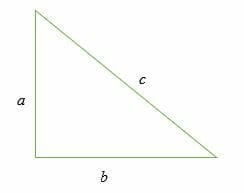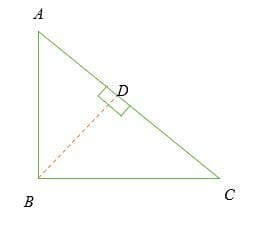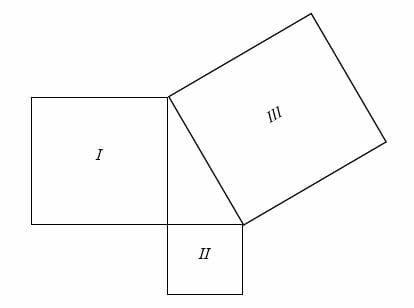# Pythagorean Theorem – Explanation & Examples

The Pythagorean Theorem which is also referred to as ‘Pythagoras theorem’ is arguably the most famous formula in mathematics that defines the relationships between the sides of a right triangle.

The theorem is attributed to a Greek mathematician and philosopher by the name Pythagoras (569-500 B.C.E.). He has many contributions to the field of mathematics, but the Pythagorean Theorem is the most important of them.

Pythagoras is credited with several contributions in the field of mathematics, astronomy, music, religion, philosophy etc. One of his notable contribution to mathematics is the discovery of the Pythagorean Theorem. Pythagoras studied the sides of a right triangle and discovered that, the sum of the square of the two shorter sides of the triangles is equal to the square of the longest side.

In this article, we will learn what the Pythagorean Theorem entails, its converse and the Pythagorean Theorem formula. Before getting deeper into the topic, let’s recall about a right triangle. A right triangle is a triangle with one interior angle equals to 90 degrees. In a right triangle, the two short legs meet at an angle of 90 degrees. The hypotenuse of a triangle is side which opposite the 90-degree angle.

## What is the Pythagorean Theorem?

The Pythagoras theorem is a mathematical law that states that, the sum of squares of the lengths of the two short sides of the right triangle is equal to the square of the length of the hypotenuse.

The Pythagoras theorem is algebraically written as:

a2 + b2 = c2## How to do the Pythagorean theorem?Consider a right triangle above.

Given that:

∠ ABC= 90°.

Let BD be the perpendicular line to the side AC.

Similar ∆s:

∆ADB and ∆ABC are similar triangles.

From similarity rule,

⇒ AD × AC = (AB) 2 —————– (i)

Similarly;

∆BDC and ∆ABC are similar triangles. Therefore;

⇒ DC/BC = BC/AC

⇒ DC × AC = (BC) 2 —————– (ii)

By combining equation (i) and (ii), we get,
AD × AC +  DC × AC = (AB) 2 + (BC) 2

⇒ (AD + DC) × AC = (AB) 2 + (BC) 2

⇒ (AC)2 = (AB) 2 + (BC) 2

Therefore, if we let AC = c; AB = b and BC = b, then;

⇒ c2 = a2 + b2

There are many demonstrations of Pythagorean Theorem given by different mathematicians.

Another common demonstration is to draw the 3 squares in such a way that they form a right triangle in between, and the area of the bigger square (the one at hypotenuse) is equal to the sum of the area of the smaller two squares (the ones on the two sides).

Consider the 3 squares below:They are drawn in such a way that they form a right triangle. Now their areas can be written in equation form:

Area of Square III = Area of Square I + Area of Square II

Let’s suppose the length of square I, square II and square III are a, b and c, respectively.

Then,

Area of Square I = a 2

Area of Square II = b 2

Area of Square III = c 2

Hence, we can write it as:

a 2 + b 2 = c 2

which is a Pythagorean Theorem.

## The Converse of the Pythagorean Theorem

The converse of the Pythagorean theorem is a rule that is used to classify triangles as either right triangle, acute triangle or obtuse triangle.

Given the Pythagorean Theorem, a2 + b2 = c2 then;

• For an acute triangle, c2< a2 + b2, where, c is the side opposite the acute angle.
• For a right triangle, c2= a2 + b2, where c is the side the 90-degree angle.
• For an obtuse triangle, c2> a2 + b2, where c is the side opposite the obtuse angle.

Example 1

Classify a triangle whose dimensions are; a = 5 m, b = 7 m and c = 9 m.

Solution

According to the Pythagorean Theorem, a2 + b2 = c2 then;

a2 + b2 = 52 + 72 = 25 + 49 = 74

But, c2 = 92 = 81
Compare: 81 > 74

Hence, c2 > a2 + b2 (obtuse triangle).

Example 2

Classify a triangle whose side lengths a, b, c is, 8 mm, 15 mm and 17 mm respectively.

Solution
a2 + b2 = 82 + 152 = 64 + 225 = 289
But, c2 = 172 = 289
Compare:289 = 289

Therefore, c2 = a2 + b2 (right triangle).

Example 3

Classify a triangle whose side lengths are given as;11 in, 13 in and 17 in.

Solution
a2 + b2 = 112 + 132 = 121 + 169 = 290
c2 = 172 = 289
Compare: 289 < 290

Hence, c2 < a2 + b2 (acute triangle)

## The Pythagoras Theorem Formula

The Pythagoras Theorem formula is given as:

⇒ c2 = a2 + b2

where;

c = Length of the hypotenuse;

a = length of one side;

b = length of the second side.

This formula can be used to solve various problems involving right angled triangles. For instance, we can use the formula to determine the third length of a triangle, when the lengths of two sides of the triangle are known.

### Application of Pythagoras Theorem formula in Real Life

• Pythagoras theorem can be used to check whether a triangle is a right triangle or not.
• In oceanography, the formula is used to calculate the speed of sound waves in water.
• Pythagoras theorem is used meteorology and aerospace to determine the sound source and its range
• Pythagoras theorem can be used to calculate the dimension of electronic components such as tv screens, computer screen, solar panels etc.
• The Pythagorean Theorem is used to calculate gradient of a certain landscape.
• In navigation, the theorem is used to calculate the shortest distance between given points.
• In architecture and construction, Pythagorean theorem can be used to calculate the slope of a roof, drainage system, dam etc.

Worked examples of Pythagoras theorem:

Example 4

The two short sides of a right triangle are 5 cm and 12cm. Find the length of the third side

Solution

Given, a = 5 cm

b = 12 cm

c = ?

From the Pythagoras Theorem formula; c2 = a2 + b2, we have;

c2 = a2 + b2

c2 =122 + 52

c2 = 144 + 25

√c2 = √169

c = 13.

Therefore, the third is equal to 13 cm.

Example 5

The diagonal and one side length of a triangular side is 25cm and 24cm respectively. What is the dimension of the third side?

Solution

Using Pythagoras Theorem,

c2 = a2 + b2.

Let b = third side

252 = 242 + b2
625 = 576 + b2
625 – 576 = 576 – 576 + b2
49 = b2
b 2 = 49

b = √49 = 7 cm

Example 6

Find the size of a computer screen whose dimensions are 8 inches and 14 inches.

Hint: The diagonal of the screen is its size.

Solution

The size of a computer screen is the same as the diagonal of the screen.

Using Pythagoras Theorem,

c2 = 82 + 152

Solve for c.

c2 = 64 + 225

c2 = 289

c = √289

c = 17

Hence, the size of the computer screen is 17 inches.

Example 7

Find the area of a right triangle, given that diagonal and the base is 8.5 cm and 7.7 cm respectively.

Solution

Using Pythagoras Theorem,

8.52 = a2 + 7.52

Solve for a.

72.25 = a2 + 56.25

72.25 – 56.25 = k2 + 56.25 – 56.25

16 = a2

a = √16 = 4 cm

Area of a right triangle = (½) x base x height

= (½ x 7.7 x 4) cm2

= 15.4 cm2

Example 8:

If the hypotenuse of a right triangle is 25 mm and its height is 17 mm less than its, find the base and the height.

Solution:

Apply the Pythagorean theorem

⇒ (x – 17)+ x= 252

Simplify.

⇒ x– 2(x) (17) + 172 + x= 625

⇒ x– 34x + 289 + x= 625

⇒ 2x– 34x + 289 = 625

⇒ 2x– 34x – 336 = 0

⇒ x– 17x – 168 = 0

⇒ (x + 7) (x – 24) = 0

⇒ x + 7 = 0

or

⇒ x – 24 = 0

⇒ x = -7, 24

Since length cannot be negative, then

⇒ x – 17 = 7

Therefore, the base is 24 mm and height, is 7 mm.

Practice Questions

1. A 20 m long rope is stretched from the top of a 12 m tree to the ground. What is the distance between the tree and the end of the rope on the ground?
2. A 13 m long ladder is leaning again the wall. If the ground distance between the foot of the ladder and the wall is 5 m, what is the height of the wall?
3. The height and base of a right triangle are 7√3 cm and 7cm respectively. Find the length of the diagonal.
4. The base and height of an isosceles triangle are 10 yards and √24 yards respectively. What is the length of the shorter side of the triangle?
5. Find the height of an equilateral triangle of side length 8 inches.